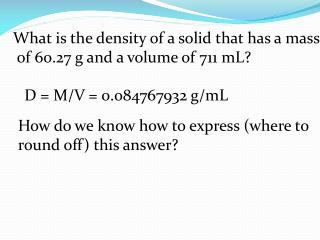DownloadDownload PresentationWhat is the density of a solid that has a mass of 60.27 g and a volume of 711 mL?

# What is the density of a solid that has a mass of 60.27 g and a volume of 711 mL?

Download Presentation## What is the density of a solid that has a mass of 60.27 g and a volume of 711 mL?

- - - - - - - - - - - - - - - - - - - - - - - - - - - E N D - - - - - - - - - - - - - - - - - - - - - - - - - - -
##### Presentation Transcript

1. What is the density of a solid that has a mass of 60.27 g and a volume of 711 mL? D = M/V = 0.084767932 g/mL How do we know how to express (where to round off) this answer?

2. Significant (Measured) Digits in Calculations All nonzero digits are significant. Evaluating Zero as a Significant Digit A zero is significant when it is : 1. Between nonzero digits: 205 61.09 2. At the end of a number that includes a decimal point: 0.500 25.160 3.00 20.

3. A zero is not significant when it is : 1. Before the first nonzero digit. (These zeros are used to locate a decimal point.): 0.0025 0.0108 2. At the end of a number without a decimal point: 1000 590

4. Practice Problem • How many significant figures are in each of • these numbers? • a) 4.5 inches e) 25.0 grams • b) 3.025 feet f) 12.20 liters • c) 125.0 meters g) 100,000 people • d) 0.001 mile h) 205 birds 2 3 4 4 1 4 3 1

5. Rounding Off Numbers Look at the next digit (after those you want to retain for the proper number of significant digits) If it is 5 or greater → round up. If it is 4 or less → round down.

6. Practice Problem • Round off these numbers to the number of • significant figures indicated: • 42.246 (four) • 88.015 (three) • 0.08965 (three) • 0.08965 (two) • e) 225.3 (three) • f) 14.150 (three) 42.25 88.0 0.0897 0.090 225 14.2

7. Multiplication or Division Round off your answer to be no more than the fewest number of significant digits.

8. Practice Problem • (134 in.)(25 in.) = ? • b) 213 miles • 4.20 hours = ? • c) (2.2)(273) • 760 = ? 3400 in2 Limited to 2 sigs 50.7 mi/hr Limited to 3 sigs .79 Limited to 2 sigs

9. Addition or Subtraction Round off the answer to no more than the fewest number of places to the right of the decimal. (i.e greatest level of shared confidence)

10. Practice Problem • How many significant figures should the • answer in each of these calculations contain? • (14.0)(5.2) • (.1682)(8.2) • c) (160)(33) • 4 • d) 8.2 + 0.125 2 sigs = 73 2 sigs = 1.4 0.125 8.2 = 8.3 1 sig = 1000 2 sigs - tenths

11. How many significant figures should the • answer in each of these calculations contain? • e) 119.1 – 3.44 • f) 94.5 • 1.2 • g) 1200 + 6.34 • h) 1.6 + 23 – 0.005 = 115.7 4 sigs - tenths = 79 2 sigs 2 sigs - hundreds = 1200 2 sigs - singles = 25